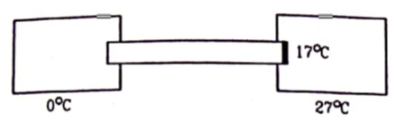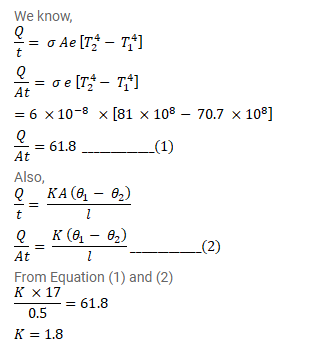# A cylindrical rod of length 50 cm and cross - sectionalQuestion:

A cylindrical rod of length $50 \mathrm{~cm}$ and cross - sectional area $1 \mathrm{~cm}^{2}$ is fitted between a large ice chamber at $0^{\circ} \mathrm{C}$ and an evacuated chamber maintained at $27^{\circ} \mathrm{C}$ as shown in figure. Only small portions of the rod are inside the chambers and the rest is thermally insulated from the surrounding. The cross - section going into the evacuated chamber is blackened so that it completely absorbs any radiation falling on it. The temperature of the blackened end is $17^{\circ} \mathrm{C}$ when steady state is reached. Stefan constant $\sigma=6.0 \times 10^{-8} \mathrm{~W} / \mathrm{m}^{2}-K^{4}$. Find the thermal conductivity of the material of the rod.Solution: